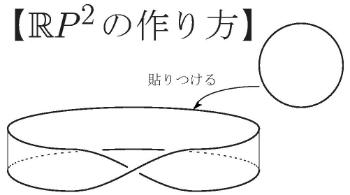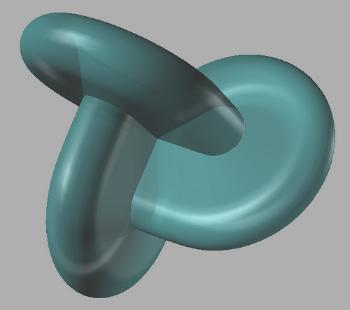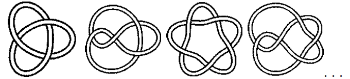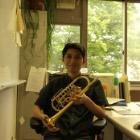Welcome to the Curious World of Science!
Research of Science at Shinshu University.

# Aspects of embeddings

## Current research theme: Aspects of embeddings

In geometry we study properties of gures. My research interest is in Topology, a branch of geometry. In particular I'm interested in gures called manifolds. All the gures in this text are manifolds.
The news that the Poincarè conjecture, one of the long-standing problems in topology, has been solved by G. Perelman was a screaming one even for non-mathematicians. It might be partially because Perelman declined Fields medal at the International Congress of Mathematics in 2006. Japanese people may remember that they saw TV program concerning Poincarè conjecture, and perhaps some people who was watching such TV programs wondered what are "4-dimensional spaces" (4-spaces for short). I'd like to encourage you to study mathematics in Shinshu University, if you do not feel rejection of "4-spaces" and rather get interested in 4-spaces.
But soon after beginning studying mathematics in　University, you will be aware that it is not so easy to understand what the mathematical problems such as Poincarè conjecture exactly means When you open a book of mathematics, you will nd only boring equations and arrows. For example, the above

$\mathbb{R}^4=\{(x_1,x_2,x_3,x_4)\mid x_i\,\text{are real numbersg}\}$

In R4 you nd no dreams nor hopes, that should be found in the 4-dimensional Pocket of Doraemon. In fact it is unavoidable; math textbooks have to be written in rigorous and non-subjective form so that they can tell the same contents to all the people. I believe you would be able to see the dreamful and hopeful 4-space, after studying hard. Below I'd like to show you how mathematicians were/are working hard to "see" higher dimensional spaces. Here I'd like to emphasis that difficulties makes mathematics fun.
I'm interested in embeddings of manifolds. Embedding an $n$-dimensional manifold ($n=1,2,3$, or higher) is to realize the manifold as a figure in the higher dimensional spaces. Embedding a manifold can be regarded as a tool to "see" the manifold. For example, consider two disks of the same radius. Gluing them along their boundaries, you obtain a (bit wrinkled) sphere, denoted by $S^2$.

## Research Area: Embeddings of manifolds

This $S^2$ can be obtained by using planar' disks, and hence this $S^2$ is a $2$-dimensional object that is realized in our $3$-space. This is an embedding of $S^2$ into $3$-space.
The surface of the earth is approximately $S^2$, but it would be natural for antient people to imagine that the ground is a plane. There are various way to know that the earth is indeed $S^2$, and perhaps one of the easiest way is to look at the earth from the Universe! The fact that the earth ($=S^2$) is embedded into the Universe, a $3$-space, enables us to `see'' the full picture of the earth.
Let's turn to a bit more complicated example. Cut out a $2\,\text{in}\times 15.7\,\text{in}$-rectangle and glue the short opposit sides after twisting the band, then you obtain a famous \emph{M\"obius band}. You see that its boundary is connected and its length is 31.4in. Glue the M\"obius band and the disk of radius 10in (the length of whose boundary is about 31.4in) along their boundaries. See Figure 1 below.If you have no supernatual power, I guess you have failed! In fact we can consider such a gluing abstractly, and the resulting figure (called the projective plane and denoted by $\mathbb{R}P^2$) is known to be never embedded into $3$-space. Both $S^2$ and $\mathbb{R}P^2$ can be obtained by gluing some planar pieces and are $2$-dimensional objects, but $\mathbb{R}P^2$ cannot be embedded into $3$-space while $S^2$ can be. This difference reflects the difference of "shapes" of $S^2$ and $\mathbb{R}P^2$.
Perhaps some people succeeded to make $\mathbb{R}P2$ even if they do not have any supernatural power. In fact it is known that $\mathbb{R}P^2$ can be realized in $3$-space if we are allowed to make "self-intersection". Such a realization is called an immersion. Figure 2 below is an immersion discovered by W.~Boy. Self-intersections make the figure confusing, but you may feel you can "see" the full picture of misterious $\mathbb{R}P^2$.One of the most important examples of embeddings is link. A link is a disjoint union of knotted circles in the $3$-dimensional space, and is an embedding of circles into $3$-dimensional space..The fact that there are infinitely many links reflects the nature of the $3$-space. Perhaps you may expect that our $3$-space is easy to understand, but in fact $3$-spaces are quite difficult. For example, the above mentioned Poincarè conjecture concerns the characterization of $S^3$, a $3$-dimansional variant of $S^2$ and the most fundamental $3$-manifold, but it has took about 100 years to solve the Poincarè conjecture (higher-dimensional analogues have been solved first). In fact the difficulties of $3$-manifolds lie in links;
Theorem (W.~B.~R.~Lickorish, A.~H.~Wallace). The classification problem of $3$-manifolds is 'essentially' equal to that of links.
This theorem is one of the reasons why the link theory is widely studied. Links and embeddings might perhaps tell us the "shape" of our Universe, one of the $3$-spaces.
As links, one manifold may admit two or more embeddings. For example there exist many way to embed $S^2$ into $4$-space (such embeddings are called $2$-links), while embeddings of $S^2$ into $3$-space are essentially unique. The classification of $2$-links is an important problem in that it relates closely to the nature of $4$-spaces, and $2$-links are also actively studied.
I'm studying the aspects of the sets of all the admissible embeddings of a fixed manifold. For example I found some deep structures of the set of embeddings of $S^3$ into $6$-spaces.
Note that I have drawn the Figures of Boy surface and links from the websites respectively "sketches of topology" and "knot atlas".}Keiichi SAKAI Mathematics